# Mathematics | Planar Graphs and Graph Coloring

Prerequisite – Graph Theory Basics
Consider an electronic circuit having several nodes with connections between them. Is it possible to print that circuit on a single board such that none of the connections cross each other i.e. they do not overlap or intersect?
This question can be answered if we know about planarity of graphs.

Planarity – “A graph is said to be planar if it can be drawn on a plane without any edges crossing. Such a drawing is called a planar representation of the graph.”

Important Note – A graph may be planar even if it is drawn with crossings, because it may be possible to draw it in a different way without crossings.
For example consider the complete graphand its two possible planar representations –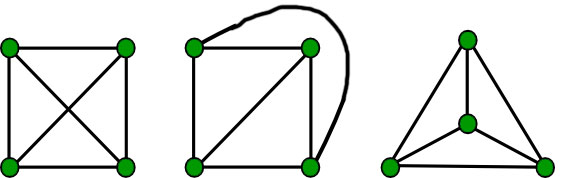• Example – Is the hypercubeplanar?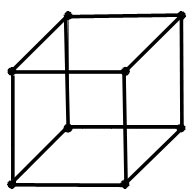• Solution – Yes,is planar. Its planar representation-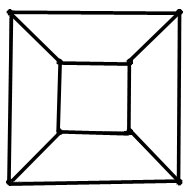Regions in Planar Graphs –

The planar representation of a graph splits the plane into regions. These regions are bounded by the edges except for one region that is unbounded. For example, consider the following graph ”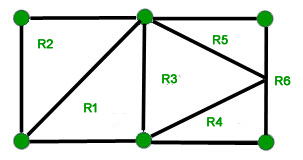There are a total of 6 regions with 5 bounded regions and 1 unbounded region.
All the planar representations of a graph split the plane in the same number of regions. Euler found out the number of regions in a planar graph as a function of the number of vertices and number of edges in the graph.

Theorem – “Letbe a connected simple planar graph withedges andvertices. Then the number of regionsin the graph is equal towhere k is the no. of component in the graph.
.”

• Example – What is the number of regions in a connected planar simple graph with 20 vertices each with a degree of 3?
• Solution – Sum of degrees of edges = 20 * 3 = 60. By handshaking theorem,which gives.
By Euler’s theorem, the number of regions =which gives 12 regions.

An important result obtained by Euler’s formula is the following inequality –

Note –“Ifis a connected planar graph withedges andvertices, where, then. Alsocannot have a vertex of degree exceeding 5.”

• Example – Is the graphplanar?
• Solution – Number of vertices and edges inis 5 and 10 respectively. Since 10 > 3*5 – 6, 10 > 9 the inequalityis not satisfied. Thus the graph is not planar.

Graph Coloring –

If you ever decide to create a map and need to color the parts of it optimally, feel lucky because graph theory is by your side. What is the maximum number of colors required to color the regions of a map? This question along with other similar ones have generated a lot of results in graph theory.
First, let us define the constraint of coloring in a formal way-

Coloring – “A coloring of a simple graph is the assignment of a color to each vertex of the graph such that no two adjacent vertices are assigned the same color.”

A simple solution to this problem is to color every vertex with a different color to get a total ofcolors. But in some cases, the actual number of colors required could be less than this.

chromatic number –“The least number of colors required to color a graph is called its chromatic number. It is denoted by.”

For planar graphs the finding the chromatic number is the same problem as finding the minimum number of colors required to color a planar graph.

4 color Theorem – “The chromatic number of a planar graph is no greater than 4.”

• Example 1 – What is the chromatic number of the following graphs?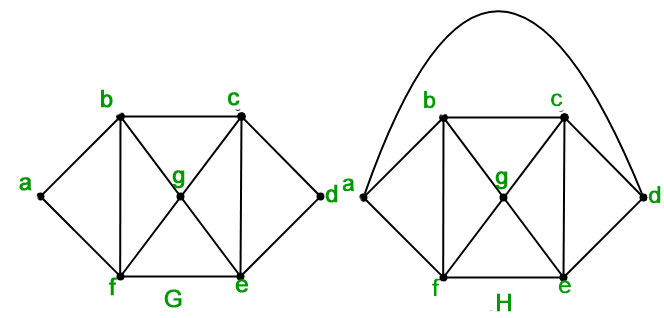• Solution – In graph, the chromatic number is atleast three since the vertices,, andare connected to each other.
The following color assignment satisfies the coloring constraint –– Red– Green– Blue– Red– Green– Blue– Red
Therefore the chromatic number ofis 3.
In graphsinceandare also connected, therefore the chromatic number if 4.
• Example 2 – What is the chromatic number of?
• Solution – Since every vertex is connected to every other vertex in a complete graph, the chromatic number is.
• Example 3 – What is the chromatic number of?
• Solution – If the vertex are colored in an alternating fashion, the cycle graph requires 2 colors. Ifis odd, then the last vertex would have the same color as the first vertex, so the chromatic number will be 3. But if it is even, then first and last vertices will be of different color and the chromatic number will be 2.
• Example 4 – What is the chromatic number of?
• Solution – In the bi-partite graph, the vertices in are divided into two sets, such that there is no edge between vertices in the same set. Therefore the chromatic number of any bipartite graph is 2. One set of vertices can be assigned one color and the other can be assigned a different color for a total of 2 colors. It will satisfy the coloring constraint since the vertices of the same set are not connected.

GATE CS Corner Questions

Practicing the following questions will help you test your knowledge. All questions have been asked in GATE in previous years or in GATE Mock Tests. It is highly recommended that you practice them.

References-

Planar Graphs – Wikipedia
Graph Coloring – Wikipedia
Discrete Mathematics and its Applications, by Kenneth H Rosen

This article is contributed by Chirag Manwani. If you like GeeksforGeeks and would like to contribute, you can also write an article using contribute.geeksforgeeks.org or mail your article to contribute@geeksforgeeks.org. See your article appearing on the GeeksforGeeks main page and help other Geeks.